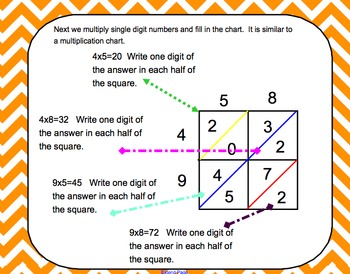# Multiplication through Thousands For Kids Who Can't MultiplySubject
File Type

NOTEBOOK (SMARTboard) File

(206 KB|17 pages)
Product Description
This is a smartboard lesson that teaches students and teachers how to multiply large numbers using the Lattice Method. I use this method in my special ed class and I have been able to teach 4th and 5th graders how to complete grade level multiplication problems without using a calculator. This is a step by step lesson for both the teacher and the student. The last few pages are different templates for using the Lattice Method that can be printed for student use.

Common Core Standards:

4.NBT.5. Multiply a whole number of up to four digits by a one-digit whole number, and multiply two two-digit numbers, using strategies based on place value and the properties of operations. Illustrate and explain the calculation by using equations, rectangular arrays, and/or area models.

5.NBT.5. Fluently multiply multi-digit whole numbers using the standard algorithm.
Total Pages
17 pages
N/A
Teaching Duration
N/A
Report this Resource to TpT
Reported resources will be reviewed by our team. Report this resource to let us know if this resource violates TpT’s content guidelines.